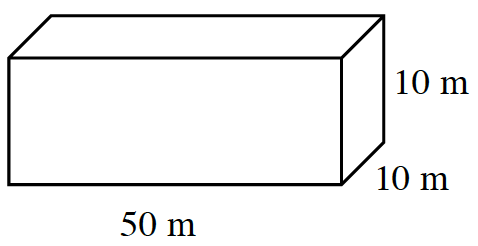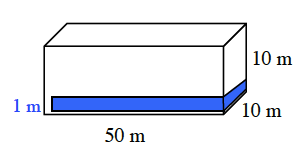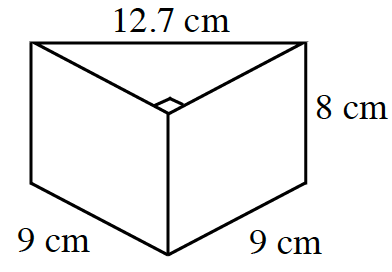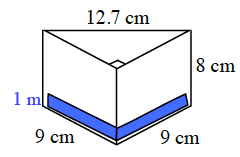### Home > ACC7 > Chapter 9 Unit 6 > Lesson CC2: 9.2.4 > Problem9-88

9-88.

Find the volume of a $1$-unit-high layer of each prism below. Then find the total volume and the surface area of each figure.

1. A rectangular prism$\text{Volume of a prism}=(\text{area of base})(\text{height})$

Consider the prism's volume in terms of multiple layers that are each one unit high and stacked depending on the height.

Solve for the volume of the prism if it had only a $1$-unit-high layer and then for the actual respective number of layers based on the height.Volume ($1$ unit layer): $(50\text{ m}·10\ \text{m})(1\ \text{m})(500\ \text{m})(1\ \text{m})=500\ \text{m}^3$
Volume (Total): $(50\ \text{m}·10\ \text{m})(10\ \text{m})(500\ \text{m})(10\ \text{m})=5000\ \text{m}^3$

1. A triangle-based prismSee part (a).